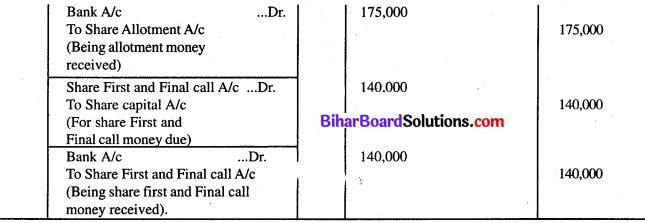# Bihar Board 12th Accountancy Model Question Paper 4 in English Medium

Bihar Board 12th Accountancy Model Papers

## Bihar Board 12th Accountancy Model Question Paper 4 in English Medium

Time : 3 Hours 15 Min
Full Marks: 100

Instructions for the candidates

1. Candidates are required to give their answers in their own words as far as practicable.
2. Figures in the right-hand margin indicate full marks.
3. While answering the questions, the candidate should adhere to the word limit as for as practicable.
4. 15 minutes of extra time has been allotted for the candidate to read the questions carefully.
5. This question paper has two sections: Section – A and Section – B.
6. In Section – A, there are 50 objective type questions which are compulsory, each carrying 1 mark. Darken the circle with black/blue ball pen against the correct option on OMR Sheet provided to you. Do not use Whitener/Liquid/Blade/Nail on OMR Sheet, otherwise, the result will be treated as invalid.
7. In Section – B there are Non-objective type questions. There are 25 Short answer type questions, out of which any 15 questions are to be answered. Each question carries 2 marks. Apart from this, there are 8 Long answer type questions, out of which any 4 of them are to answer. Each question carries 5 marks.
8. Use of any electronic device is prohibited.

Objective Type Questions

Question No. 1 to 50 have four options, out of which only one is correct, you have to mark the correct option on the OMR Sheet. (50 × 1 = 50)

Question 1.
Life membership fees received by a club is shown in
(a) Income & Expenditure A/c
(b) Balance Sheet
(c) Profit & Loss A/c
(d) None of these
(b) Balance Sheet

Question 2.
Receipts and- Payments Account is a
(a) Personal A/c
(b) Real A/c
(c) Nominal A/c
(d) None of these
(b) Real A/cQuestion 3.
Payment of honorarium to the secretary is treated as
(a) Capital Expenditure
(b) Revenue Expenditure
(c) Cash Expenses
(d) None of these
(b) Revenue Expenditure

Question 4.
Outstanding subscription is a/an
(a) Income
(b) Asset
(c) Liability
(d) None of these
(b) Asset

Question 5.
Which of the following is not an income?
(a) subscription
(b) Donation
(c) Sale of ticket
(d) Endowment fund
(b) DonationQuestion 6.
Receipts and Payments Account usually indicates.
(a) Surplus
(b) Capital fund
(c) Debit balance
(d) Credit balance
(b) Capital fund

Question 7.
Income and Expenditure Account generally indicates
(a) Surplus/deficit
(b) Cash Balance
(c) Capital Fund
(d) Net Profit/Loss
(a) Surplus/deficit

Question 8.
In the absence of partnership deed, the partner will be allowed interest on the amount advanced to the firm
(a) @5%
(b) @6%
(c) @7%
(d) @8%
(b) @6%

Question 9.
Which one is not the feature of partnership?
(a) Agreement
(b) Profit-sharing
(c) Limited Liability
(d) Two or more persons.
(d) Two or more persons.

Question 10.
In the absence of partnership deed, interest on capital will be given to the partners at
(a) 8% p.a.
(b) 6% p.a.
(c) 9% p.a.
(d) None of these
(d) None of theseQuestion 11.
The Current Account of the partners will be
(a) Debit Balance
(b) Credit Balance
(c) Both
(d) None of these
(c) Both

Question 12.
Interest on partners capital is calculated on
(a) Capital in the beginning
(b) Capital at the end
(c) Average capital
(d) None of these
(b) Capital at the end

Question 13.
Which of the following is an appropriation of profit?
(a) Interest on loan
(b) Interest on capital
(c) Salary
(d) Rent
(b) Interest on capital

Question 14.
In the absence of any partnership agreement, the profits & losses of the firm are divided into
(a) capital ratio
(b) equal ratio
(c) any of these two ratios
(d) none of these
(b) equal ratioQuestion 15.
In an ordinary partnership, the maximum number of partners is
(a) 20
(b) 10
(c) 15
(d) 5
(a) 20

Question 16.
In the absence of a partnership deed, partners are not entitled to receive
(a) Salaries
(b) Commission
(c) Interest on Capital
(d) All of these
(d) All of these

Question 17.
The balance of Revaluation Account is transferred to old partners Capital A/c in there
(a) Old Profit or loss Sharing ratio
(b) New profit or loss sharing ratio
(c) Equal ratio
(d) none of these
(a) Old Profit or loss Sharing ratio

Question 18.
Revaluation Account is a
(a) Personal Account
(b) Real Account
(c) Nominal Account
(d) None of these
(c) Nominal AccountQuestion 19.
Sacrifice ratio is
(a) New ratio – Old ratio
(b) Old ratio – New ratio
(c) Gaining ratio – Old ratio
(d) Old ratio – Gaining ratio
(a) New ratio – Old ratio

Question 20.
Goodwill is
(a) Tangible Asset
(b) Intangible Asset
(c) Current Asset
(d) None of these
(b) Intangible Asset

Question 21.
The executors of a deceased partner will be paid interest on the amount due from the date of death of the partner at
(a) 5% p.a.
(b) 6% p.a.
(c) 7% p.a.
(d) 8% p.a.
(b) 6% p.a.

Question 22.
Share of goodwill brought by a new partner in cash is shared by old partners in
(a) Sacrifice ratio
(b) Old ratio
(c) New ratio
(d) All of these
(c) New ratio

Question 23.
Balance Sheet prepared after new partnership agreement, assets and liabilities a recorded at
(a) Original value
(b) Revalued figure
(c) Realisable value
(d) Either of (a) or (b)
(b) Revalued figureQuestion 24.
Which of the following assets is compulsorily revalued at the time of admission of a new partner?
(a) Stock
(b) Fixed Assets
(c) Investment
(d) Goodwill
(d) Goodwill

Question 25.
Share Allotment Account is
(a) Personal Account
(b) Real A/c
(c) Nominal A/c
(d) None of these
(a) Personal Account

Question 26.
Equity shareholders are
(a) Customers
(b) Owners
(c) Creditors
(d) Debtors
(b) Owners

Question 27.
The total amount of liabilities side includes
(a) Authorised Capital
(b) Issued Capital
(c) Paid-up Capital
(d) Subscribed capital
(c) Paid-up CapitalQuestion 28.
Shareholders get
(a) Interest
(b) Dividend
(c) Commission
(d) Profits
(b) Dividend

Question 29.
Interest on calls in arrears is charged according to table ‘F’ at
(a) 5% p.a.
(b) 6% p.a.
(c) 7% p.a.
(d) 8% p.a.
(d) 8% p.a.

Question 30.
Revaluation A/c is prepared at the time of
(b) Retirement
(c) Death
(d) All of these
(d) All of these

Question 31.
Which one of the following is the registered capital of the company?
(a) Paid-up capital
(b) Uncalled up capital
(c) Authorised capital
(d) Issued capital
(c) Authorised capital

Question 32.
Debenture is a
(a) Loan certificate
(b) Cash certificate
(c) Credit certificate
(d) None of these
(a) Loan certificateQuestion 33.
Debenture holders are the
(a) Customers
(b) Owners
(c) Creditors
(d) Debtors
(c) Creditors

Question 34.
Rate of interest on debentures is at
(a) 12% p.a.
(b) 20% p.a.
(c) fixed rate
(d) 15% p.a.
(c) fixed-rate

Question 35.
Debentures can be redeemed out of
(a) Profit
(b) Capital
(c) Provision
(d) All of these
(d) All of these

Question 36.
The premium on redemption of debentures is a
(a) Personal A/c
(b) Real A/c
(c) Nominal A/c
(d) Suspense A/c
(b) Real A/cQuestion 37.
The premium on redemption of Debenture A/c is
(a) Asset
(b) Expense
(c) Liability
(d) Income
(b) Expense

Question 38.
The Current Assets include
(a) Stock
(b) Debtor
(c) Cash
(d) All of these
(d) All of these

Question 39.
The dividend is usually paid on
(a) Authorised Capital
(b) Issued Capital
(c) Paid-up capital
(d) Called-up capital.
(c) Paid-up capital

Question 40.
Interest on loans is
(a) Operating Expense
(b) Direct Expense
(c) Indirect Expense
(d) All of these
(c) Indirect Expense

Question 41.
Interest on calls in arrears is charged according to ‘Table A’ at
(a) 5%p.a.
(b) 6% p.a.
(c) 8% p.a.
(d) 11% p.a.
(a) 5%p.a.Question 42.
Realisation Account is a
(a) Personal A/c
(b) Nominal A/c
(c) Real A/c
(d) None of these
(c) Real A/c

Question 43.
Expenses on the dissolution of the firm are called
(a) Realisation expenses
(b) Legal expenses
(c) Loss Expenses
(d) None of these
(a) Realisation expenses

Question 44.
X, Y and Z share profits in the ratio of 1/2 : 1/3 : 1/6. Z dies, New ratio of X and Y will be
(a) 3 : 2
(b) 2 : 3
(c) 2 : 1
(d) None of these
(a) 3 : 2Question 45.
The executors of a deceased partner will be a partner will be paid interest on the amount due from the date of death of the partner at
(a) 5% p.a.
(b) 6% p.a.
(c) 7% p.a.
(d) 8% p.a.
(b) 6% p.a.

Question 46.
A and B share profits and losses in the ratio of 3 : 1. C is admitted into a partnership for 14 shares. The sacrificing ratio of A and B is
(a) equal
(b) 3 : 1
(c) 2 : 1
(d) 3 : 2
(b) 3 : 1

Question 47.
A, B and C are equal partners in the firm. B retires and the remaining partners decide to share profits of the new firm in the ratio of 5 : 4. Gaining ratio will be
(a) 2 : 1
(b) 1 : 2
(c) 4 : 5
(d) 5 : 4
(a) 2 : 1Question 48.
A, B and C are sharing profits in the ratio of 1/2 : 1/3 : 1/6. C retired. Gaining ratio will be
(a) 2 : 1
(b) 2 : 3
(c) 3 : 2
(d) 1 : 2
(c) 3 : 2

Question 49.
The decrease in the value of fixed assets is termed as
(a) Depreciation
(b) loss
(c) profit
(d) expense
(a) Depreciation

Question 50.
The excess of average profits over the normal profits are called
(a) Super profits
(b) fixed profits
(c) abnormal profits
(d) normal profits
(a) Super profits

Non-Objective Type Questions

Question No. 1 to 25 are Short answer type questions. Answer any 15 out of them. Each question carries 2 marks. (15 × 2 = 30)

Question 1.
Explain the reason for the valuation of goodwill.
Steps for valuation of Goodwill are shown below.
Steps Involved under Average Profit method.
Step 1: Calculate Adjusted (past) Profits or maintainable profit for each of the relevant years (given) after making adjustments relating to abnormal gains, profits, abnormal expenses and losses, incomes from non-trade investments, Proprietor’s remuneration etc.
Step 2: Calculate total profit by adding each relevant year’s adjusted profits.
Step 3: Calculate average profits:
Formula: Average profits = $$\begin{array}{l} \text { Total Profit } \\ \text { No. of Years } \end{array}$$
Step 4: Calculate Goodwill as under:
Goodwill = Average profit × No. of years purchase.

Question 2.
X, Y and Z are partners sharing profits in the ratio of 4 : 3 : 2. Y retires. X and Z decided to share profits and losses in future in the ratio of 5 : 4. Calculate gaining ratio.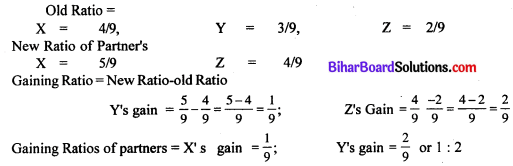Question 3.
What are the objectives of a financial statement?
Following are the objectives of financial statement: The basic objective of financial statements is to assist in decision making. But there many more other objective too.

• To serve as a media of information regarding profitability and financial health of the enterprise.
• To assist in investment decision making.
• To help in making planning.
• To help management in assessing the efficiency of the organisation.
• To provide financial information about changes in net resources arising out of business activities.
• To provide reliable information about economic resources and the obligation of business enterprise.
• To assist in estimating the earning potentials of the business.
• To disclose other information that is relevant to the needs of the users of the financial statements.Question 4.
A firm’s current ratio is 2.5 : 1 Its liquid ratio is 1.5 : 1 If current liabilities are Rs. 15,000, calculate the amounts of current assets and stock.
Calculation of current assets and stocks: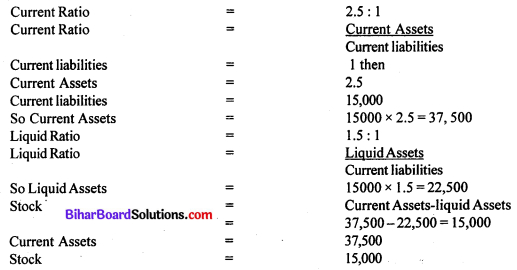Question 5.
What is Ratio Analysis? Explain the importance of Analysis.
Ratio Analysis: Ratio analysis means examination and interpretation of the numerical relationship of two numbers. In financial analysis, a ratio is used as an index or yardstick for evaluating the financial position and performance of a firm According to Myers, Ratio analysis is a study of the relationship among the various financial factors in business.
Importance of Accounting Ratios:

• Accounting ratios make the figures simple and understandable.
• Accounting ratios provide the basis for preparing budgets and also determining the future line of action.
• Accounting ratios facilitate comparative analysis of the performance.
• Accounting ratios help in making decisions from the information provided in the financial statements.
• Accounting Ratios indicate efficiency and the profitability of the business concern.
• The operational efficiency of the business can be ascertained by calculating the operating ratio.

Question 6.
Explain the importance of cash flow statement.
Importance and uses of cash flow statement:

• Cash flow statement aims at highlighting the cash generated from operating activities.
• Cash flow statement helps in planning the repayment of the loan schedule and replacement of fixed assets.
• Cash is the centre of all financial decisions. It is used as the basis for the projection of future investment and financial plans of the enterprise.
• Cash flow statement helps in the efficient and effective management of cash.
• The management generally looks into the cash flow statement to understand the internally generated cash which is the best utilised for payment of dividends.
• Cash flow statement is useful as a tool of historical analysis.Question 7.
Explain the types of cell references in Excel.
Types of cell address in excel:

• Relative cell address: A Relative cell reference is one chat when copied from one position to another will adjust the formula cell address to suit the position it is in.
• Absolute cell Address: absolute and relative references are extremely important in Microsoft excel and they ensure that the formulas you are creating actually refer to the correct cells.
• Mixed cell Address: Essentially what this means is that one either the column or the row has the dollar symbol for example & C3.

Question 8.
Define DBMS.
Database Management system (DBMS): A DBMS is a database program. Technically speaking it is a software system that asses a standard method of cataloguing, receiving, and running queries on data.

Question 9.
Explain the function of SQL command Create & Select.

• Create: Creates a user-defined function in SQL Server and Azure SQL Database.
• Select: It means to select any file and then proceed further work in SQL Database.

Question 10.
Describe various uses of MS-Excel in accounting.
Following are the various uses of Ms-Excel in Accounting:

• Select the current column
• Select the current row
• Resize the name box
• Activate Excel’s Ribbon
• View a macro within your workbook
• Subtraction, Summation, Dividing, multiplication
• Navigate the charts and other objects in a workbook.Question 11.
Define types of SQL (DML & DOL.)
Navigate to an existing name range:

1. DDL (Data Definition Language)
2. DML (Data Manipulation Language)
3. DQL (Data Query Language)
4. DCL (Data Control Language)
6. TCC (Transaction Control Command)

Question 12.
Explain Database normalization
Database normalisation is a process used to organize a database into tables and columns. The idea is that a table should be about a specific topic and that only those columns which support that topic are included. For example, a spreadsheet containing information about salespeople and customers serves several purposes.

Question 13.
Explain Entity, Attribute and Relation.

• Entity: All entities have some attributes or properties that give them their identity.
• Attribute: An attribute is a property of a given entity, for example, Roll No. is a property of entity Student. An attribute instance is a particular property of an individual entity instance.
• Relation: The types of relationship between two entities are represented in E/R diagrams by a certain symbol. An entity may be associated with one none or occurrences of another entity.

Question 14.
Explain the logical function if () in Excel with example.
IF: Tests a user-defined condition and returns one result if the condition is TRUE and another result if the condition is FALSE.
Ex. Formula = If (Logical – test, value – if – true, value – if – false.)Question 15.
Describe in excel the Goal seeks under the what-if analysis tool.
By using what: If analysis tools in Microsoft office excel, you can use several different sets of values in one or more formulas to explore the various results.
Goal seeks works with only one variable input value if you want to determine more than one input value, for example, the loan amount and the monthly payment amount for a loan you should instead use that solver add-in.

Question 16.
Describe the different processes of chart generation in excel.
Process of chart generation in Excel:

• Step 1: Start Excel by default a blank worksheet opens.
• Step 2: Select the “Insert” tab.
• Step 3: Choose process in the list of chart types.
• Step 4: Add text to the first step in the process.
• Step 5: Customize the appearance of the flow chart.
• Step 7: Click the “Save” button on the quick access toolbar or press “ctreates” to save the completed business process flow chart before closing the worksheet.

Question 17.
Explain any three financial functions of excel.
Three Financial Function of Excell:

• Present value (PV) equivalent to the value of the load or savings today, this function is used to estimate the loan size that could be paid off provided a periodic payment over a provided total number of payments and a provided periodic interest rate.
• Interest rate per period (RATE) equivalent to the interest rate generally monthly. This function is used to calculate the periodic interest rate required to pay off a provided present value with a provided periodic payment and a total number of payments.
• The number of payment periods (NPER) equivalent to the number of payment periods. This function is used to evaluate the total number of payment periods required to pay off a given present value with a provided payment and periodic interest rate.

Question 18.
Write the appropriate SQL command to create an invoice table with required columns.

• For other objects, it is not so easy to come up with an equivalent database table structure.
• Consider an invoice each invoice object contains a reference to a customer object.
• You right consider simply entering all the customer data into the invoice tables.Question 19.
Explain the function of Auto filter & sort in excel.
Auto Filter: Auto filter is an easy way to turn the values in Excel column into specific filters based on the cell content.
Sort: When you enter data into your worksheet it is often unorganised making it difficult to examine. When analyzing the information in your spreadsheet, you may need to rearrange the data in different ways to answer different questions. Excel’s sorting features can help you rearrange your data so you can use it more efficiently.

Question 20.
Subscription received during the year 2013, Rs. 30,000. Subscription Rs. 20,000 was outstanding at the end of the previous year. Calculate the income from a subscription for the year 2013.
Subscription for the year of 2013 – Rs. 30,000
Less: O/S at the end of the previous year – Rs. 20,000
Subscription for the year – Rs. 10,000

Question 21.
What is Profit and Loss Appropriation Account?
Profit and Loss Appropriation AccountProfit and loss Appropriation account is an account which is prepared to carry out adjustments of partners rights and obligations such as salary payable to partners, commission, interest on capital interest on drawings and distribution of profit among partners. It is an extension of the profit and loss account. Net profit as shown by the profit and loss account is brought down to this account.

Question 22.
Ram and Rahim are partners. Ram invested Rs. 2,00,000 and Rahim Rs. 2,50,000. In the absence of partnership, deed distributes the profit Rs. 50,000 to each partner.
In the absence of Partnership deed Profits are divided in equal ratio, therefore,
Share of Ram and Rahim – 25,000 each.Question 23.
What is meant by reconstitution of a partnership firm?
The partnership is created by an agreement, whenever, there is a change in the existing agreement, it amounts to the reconstitution of the Partnership firm.

Question 24.
What do you mean by normal profit and super profit?
Normal profit means a reasonable return on capital employed, i.e. profit earned by a similar firm in the same industry, whereas super profit means the excess of actual profit over normal profit.

Question 25.
Rajratan and Mrityunjay who share profit in the ratio of 3 : 2 admit Shashi to give him $$\frac{1}{3}$$ share. Calculate the new profit & loss sharing ratio.
Profit sharing Ratio of Rajratan and Mritunjay = 3 : 2
Shashi’s share in firm = $$\frac{1}{3}$$
Let total pforit of firm = 1;
Remaining balance of profit = $$1-\frac{1}{3}=\frac{2}{3}$$
therefore, new ratio
Rajratan = $$\frac{2}{3} \times \frac{3}{5}=\frac{6}{15}$$
Mrityunjay = $$=\frac{2}{3} \times \frac{2}{5}=\frac{4}{15}$$
Shashi = $$\frac{1}{3} \times \frac{5}{5}=\frac{5}{15}$$
New Ratio of firm = 6 : 4 : 5Question no. 26 to 33 are Long answer type questions. Answer any 4 of them. Each question carries 5 marks. (4 × 5 = 20)

Question 26.
Distinguish between Sacrifice Ratio and Gaining Ratio.
Distinguish between Sacrifice Ratio and Gaining Ratio: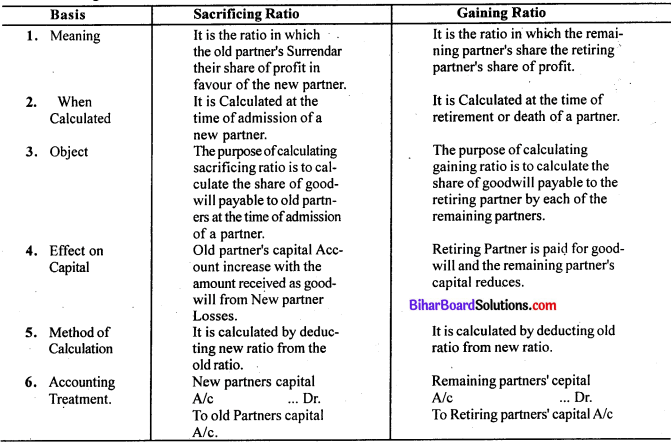Question 27.
How will you deal with the realisation expenses of the firm of Rashmi and Bindu in the following cases:
(i) Realisation expenses Rs. 1,00,000
(ii) Realisation expenses of Rs. 30,000 and paid by Rashmi.
(iii) Rashmi bears Realisation expenses for which she will be paid Rs. 70,000. The actual expenses incurred by Rashmi Rs. 1,20,000.

Journal EntriesQuestion 28.
Distinguish between preference share and equity share.
Following are the differences between preference shares and equity shares.

 Preference Shares Equity Shares 1. Preference shares have preferential right to get dividend before any dividend paid on equity shares. 1. Dividend on equity shares is paid after the payment of preference dividend and that too if there is surplus. 2. The rate of dividend on preference share is fixed. 2. The rate of dividend is not fixed in case of equity shares. 3. Preference shareholders have no right to participate in the management of the company. 3. equity shareholders have full right to participate in the management of the company. 4. Preference shareholders can vote only in special circumstances. 4. Equity shareholders have full right to vote in all circumstances. 5. In the case of cumulative preference shares arrears of dividends are accumulated, arise. 5. Accumulation of arrears of dividend on equity shares does not

Question 29.
Distinguish between shares and debentures.
Following are the differences between share and debentures.

 Shares Debentures 1. Share is a symbol of ownership. It represents a portion of the capital. 1. A debenture is the symbol of loan. It represents the debt of a company. 2. It is ownership security. 2. It is creditorship security. 3. A shareholder enjoys the voting right. 3. A debenture holder does not have voting right. 4. The dividend is paid on shares. It is an appropriation of profits. 4. Interest is paid on debentures. It is a charge against profit. 5. Payment of dividend is made only when there is a profit. There is no fixed rate of dividend on equity shares. 5. Interest on debentures is a compulsory payment whether the company earns a profit or not. 6. A share is unsecured. 6. A debenture is secured on the fixed or floating charge.Question 30.
Urbashi Ltd. purchased an established business for Rs. 50,000 payable Rs. 15,000 in cash and the balance by the issue of 9% debentures of Rs. 100 at a discount of 10%. Show journal entries.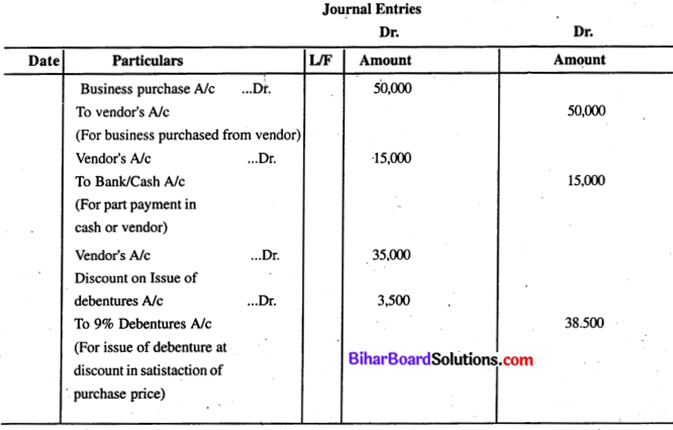Question 31.
On 31st March 2013 Puja Ltd redeemed Rs. 2,50,000. 10% debentures out of profits. Show the Journal entries.

In the books of Pooja LtdQuestion 32.
Rudra Company Ltd. issued 35000 equity shares of Rs. 10 each at a premium of Rs. 2 payable as follows:
On application – Rs. 3
On allotment – Rs. 5 (including premium)
The issues were fully subscribed and all the money were duly received Record necessary Journal entries.